# Telecommunications - X.25 Link Layer

X25 - Level2

X25 - Level2 - frame types

# 1 - The link layer

X.25 is a multi-layer protocol

It provides the following functions:

• Error detection and correction
• Division of data stream into frames

Link layer - could also be called, frame level, link level, frame layer, level 2

At the link level each 'hop' through the network is independent. that is -

If computer A has a packet to send to computer Bcomputer A 'wraps' packet into frame and sends it to PSE c. PSE c 'unwraps' the packet and decides where to send it - say PSE d, PSE c 'wraps' the packet in a completely new frame and sends it to PSE d this continues until the packet reaches computer B so the link header and trailer bytes will be different for each 'hop'

• Strictly, standards such as X.25 only define the protocol between the network and customers computer, however in practice, the link level between two Packet Switching Exchanges will be similar or identical to X.25 level 2.
• The link layer contains no routing information that is all handled by the packet layer - all the link layer does is safely transfer the packet across the link.
• Any protocol events at the link level - e.g. disconnect or flow control affect all calls on the link.

The link layer provides the following services:

• Byte synchronization
• Dividing the datastream into packets
• Error detection and recovery

## 1.2 - THE DATA-LINK LAYER

The data link layer provides functional and procedural means to establish, maintain and release data-link-connections (BRING THE LINK UP AND DOWN) among network-entities (CUSTOMERS AND SWITCHES) and to transfer data-link-service-data-units (PACKETS). A data-link connection is built upon one or several physical connections.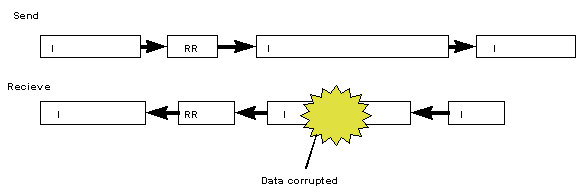## 1.3 - FUNCTIONS OF THE DATA LINK LAYER

The data-link converts the unreliable datastream into a resilient, error free link. The link may be between the terminal equipment and the network (X.25), or between adjacent nodes in the network.

It breaks up the stream of bits into blocks ("frames") of a reasonable size and adds some checking mechanism ("frame check sequence"). A copy of the frame is held at the sending end and if the receiver does not acknowledge the frame it is retransmitted.

 Protocol defined by type Bi-Sync Binary Synchronous communications IBM International Business Machines Half Duplex Polling character set based (EBCDIC or ASCII) SDLC Synchronous data link control IBM International Business Machines Half Duplex Polling independent of character set HDLC High level data link control ISO International Standards Organization NRM Normal Response Mode (=SDLC) ABM Asynchronous Balanced Mode X.25 (level 2) ITU-T (CCITT) LAP Link Access protocol LAPB Link Access protocol balanced

# Level 2 X.25

## Level 2 frame structure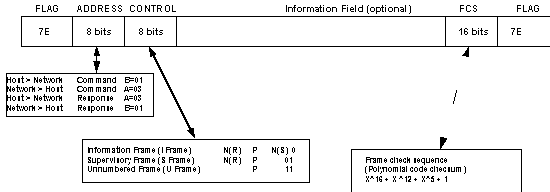# 2 - History of data-link protocols

• Bi-sync - Binary Synchronous Communications IBM (International Business Machines) protocol byte-orientated - sees the data as 8-bit bytes. use special characters for control purposes committed to specific character codes (not transparent). based on polling -central host polling remote terminals
• SDLC - Synchronous Data Link Control, IBM protocol bit-oriented - sees the data as bits like Bi-sync based on polling.
• HDLC - High Level Data link Control, ISO (international standards organization) protocol developed from SDLC but has large number of options -

 NRM normal response mode (as SDLC) ARM asynchronous response mode ABM asynchronous balanced mode

 ISO 3309 HDLC Frame structure ISO 4335 HDLC Elements of protocol ISO 6159 HDLC - Elements of procedure - unbalanced classes ISO 6256 HDLC - Elements of procedure - balanced classes

* X25 Level 2 - ITU-T CCITT (Consultative Committee for International telegraphy and telephony) protocol

protocols:

LAP (obsolete)

LAP-B is compatible with the ISO balanced classes of

procedure (class BA) with all options determined,

## Synchronization

### Bit Synchronization

Bit synchronization is derived by the modem from the incoming data stream.

There is a option in SDLC called Non Return to Zero Inverted (NRZI), this is designed to ensure that there are plenty of bit transitions in the data to help with bit synchronization, this is not required by modern modems and is not supported by Public Network X.25 or SPAD.## 3.2 - Byte Synchronization

• Frames are separated by at least one flag which is 01111110, this is '7E' in hexadecimal
• The sequence 01111110 could occur by chance in the data being sent which would cause a false flag to be detected. To stop this happening an extra zero is inserted after any sequence of five ones and this extra zero is removed at the distant end. This is known as 'bit stuffing'.
Byte synchronization is how the receiver knows where, in the serial datastream, one character stops and the next one starts.
• In Asynchronous this is done by stop and start bits, this is simple but inefficient.
• In Bisync it is done by the SYN character, this means that the SYN character cannot be used in normal data without using a special protocol.
• In SDLC/HDLC/X.25 it is done by sending a flag: Any sequence of six ones with a zero on either side is a flag - e.g. flag = 01111110 = 'hex 7E'
• The flag has a dual purpose, it provides byte synchronization and provides a marker of the beginning and end of a frame, so between frames there must be one or more flags.
• When there are no frames to send then flags are normally sent continuously the only exception is IBM SDLC which sometimes stops sending flags between frames.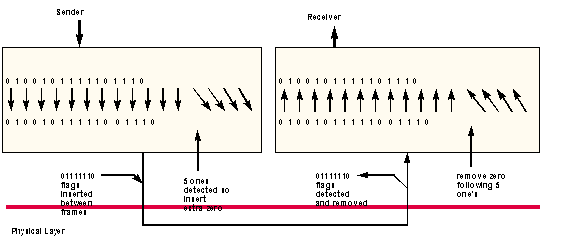# 4 - Data transparency

One of the problems of any protocols is "data transparency". That is, the user wishes to send an arbitrary data stream but the protocol itself needs to use control characters which could occur within that data stream.

There are a number of solutions to this problem:-

• Do not use some characters for data, eg. ASCII, EBCDIC codes
• Data Link Escape (DLE) (Character stuffing) - A special character DLE is used to indicate that the following character is to be interpreted as a control character. Thus if ETX ("end of text") is encountered in a block, not preceded by DLE, it is taken to be part of the data. If the sequence DLE ETX is found, then it is taken to indicate the end of text. If two DLEs are found, then it is assumed that only one DLE was in the data.
• Count Field Mechanism-Used in DDCMP (Digital Data Communication Message Protocol) PROBLEM - If the count field is corrupted then this could lead to serious inefficiencies so, for this reason, protocols using a count field nearly always have a checksum appended to the block header in addition to the ordinary checksum at the end of the block.
• Bit-Stuffing - The data-stream is considered to be a sequence of bits with no arbitrary division into bytes. If the sender wishes to send a sequence of bits containing (at least) five consecutive "1" bits, it inserts a "0" bit after the five "1"s. Similarly, if the receiver detects five "1" bits followed by a "0", it removes the zero. Such an algorithm can very easily be implemented in hardware and is very efficient.

This transparency mechanism then allows sequences of more than five "1"s to be used for control purposes and bit-oriented protocols use the sequence "01111110" as a "flag" to delimit frames (and to synchronize sender and receiver).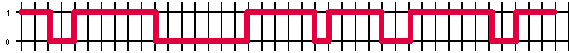# 5 - Example 2 - flags and bit insert/delete

Analyzing directly from the bits on the line is not something you will need to do in practice because all protocol testers do this automatically.

On the protocol tester you will see the data with bit-stuffing removed, so skip these examples unless you are interested.

Here is another example worked through

0100110111111011000000111110100110110100011011101111110110

----><------> ^ <------><---

end of this a zero flag start

last pattern following of next

frame must be five ones frame

a flag must have

wherever been inserted

it occurs for transparency

so remove it

this leaves the following,

1100000011111 1001101101000110111

divide up into bytes,

11000000 11111100 11011010 00110111

<---------------->

the sixteen bits

before the flag is

always the frame

check sequence

<------>

this is the

control byte

the LSB is

transmitted

first so it

is 3F in hex

which identifies

this as a SABM

frame.

<------>

this is

03 in hex

which is

Idle channel state - more than 15 ones

The action to be taken by a DCE upon detection of the idle channel state is not defined at this time.

active channel state - sending a frame or continuous flags

Disconnected phase - Set on DISC-UA exchange or expiry of timer

T3. Currently Public Network polls with DISC frames in this state.

Information phase - Set by SARM-UA, SABM-UA, SABME-UA etc.

* when no data - interframe time fill - continuous flags

- known as - active channel state

- useful test - flags shows RX line OK# 7 - Frame structure

All transmissions on a X.25/HDLC/SDLC link are organized in the following form which is known as a frame.

 Flag address 8 bits control 8 bits Information n bits FCS Flag 16 bits
• Note 1: Some recommendations define modulo 128 operation but Public Network does not support these. When modulo 128 is used 16 bit control field is used for frame formats that contain sequence numbers.
• Note 2: The information field is only used in some frame types. In the case of I-frames the length of the field is not restricted by the link layer, although restrictions may be imposed by higher layers in the protocol, e.g. maximum length or integral number of bytes.
• Note 3: Addresses and control fields shall be transmitted with the low-order bit first (i.e. the units column is transmitted first).

LAPB

In HDLC/X.25 either end of the link can initiate a command, this is faster than SDLC/HDLC NRM polling protocols, there is no delay while the DTE waits for a poll, multidrop operation cannot be used on X25.

 Command DCE to DTE Command DTE to DCE Response DCE to DTE Response DTE to DCE single link operation: A = 03 B = 01 B = 01 A = 03 multi link operation: C = 0F D = 07 C = 07 D = 0F

Slide LNK7 Mk2 - Frame types

# 9 - Control Field

This is an 8-bit field for Modulus 8 working or a 16-bit field for Modulus 128 working, this field identifies the frame type.

all done with 9 types of frame :

• Information frame - does the work
• RNR = Receiver not ready - ack information frame - puts on busy
• REJ = Reject - ack upto sequence number - reject frames sent since
• SABM = set asynchronous balanced mode - link up
• DISC = disconnect - link down
• UA = unnumbered acknowledgment - ack SABM or DISC
• FRMR = frame reject - report various errors
• DM = disconnect mode - same as UA but reports down state

## 9.1 - The P/F bit

All frames have a P or F bit in the control field called poll bit (P-bit) in commands final bit (F-bit) in responses

In SDLC or HDLC(NRM) the P/F bit is used to "turn the line round" for half-duplex working

In X.25 LAPB The P bit is used in commands to request a response from the distant end, examples of this can be seen later when polling and in error recovery.

The F-bit is used in responses only when responding to a command with the P-bit set

Slide LNK 8 Slide LNK 8 Slide LNK 8

un-used frames | | |

(or HDLC/SDLC) | | | xxx x 101 1

| SRJ selective reject | xxx x 110 1

| *UI unnumbered c or r with infomation| 000 x 001 1

| *UP unnumbered poll | 001 x 001 1

| *SNRM set normal response mode | 100 x 001 1

| | | 101 x 001 1

| | | 110 x 001 1

| *TEST | | 111 x 001 1

| *RIM | *SIM | 000 x 011 1

| | | 001 x 011 1

| | | 010 x 011 1

| | | 011 x 011 1

| | | 011 x 011 1

| | | 101 x 011 1

| *CFGR configure | | 110 x 011 1

| | | 111 x 011 1

| | | 010 x 111 1

| *SABME | | 011 x 111 1

| *RSET | | 100 x 111 1

| *XID exchange ID | | 101 x 111 1

| *SNRME | | 110 x 111 1

| *BCN beacon | | 111 x 111 1

\ 4 0 0 0 0 1 1 1 1

\ 3 0 0 1 1 0 0 1 1

\ 2 0 1 0 1 0 1 0 1

876 -------------------------------------------------------------

000 RR *UI RNR *RIM/SIM REJ *SRJ SARM

001 RR *UP RNR REJ *SRJ SABM

010 RR DISC RNR REJ *SRJ

011 RR UA RNR REJ *SRJ

100 RR *SNRM RNR FRMR REJ *SRJ *RSET

101 RR RNR REJ *SRJ

110 RR RNR *CFGR REJ *SRJ

111 RR *TEST RNR REJ *SRJ *BCN

# 10 - Information Field

In the case of I-frames the information field contains the packet level information, this is the point of the whole link layer - to get this information across the link safely, the contents of this field are covered in the packet layer.

In the case of FRMR frames the information field contains 3 bytes which contain information about the reason for the reject.

There is no theoretical maximum length of the I-field, the practical maximum length will depend on the error rate, if the error rate is high and the frames are long the retransmission overhead will become unacceptably high.

Parameter N1 defines the maximum length of the I-field, also the packet level allows a maximum length for packets to be negotiated. Some networks including Public Network require that the I-field contain an integral number of bytes although there is nothing in the protocol that requires this.

length of I-field

X.25 allows any number of bits up to max specified by packet level, some networks require the user data field to contain an integral number of octets.

slide LNK12 - error detection

11 - FCS field - Error detection and recovery

During transmission bits can be corrupted due to causes such as Gaussian (ie random) noise, thunderstorms at Reading exchange etc. These bit corruption's tend to happen in bursts, for example, a 10ms noise burst induced from electromechanical devices in a telephone exchange could corrupt 96 bits at 9600 baud.

The link layer needs to provide error free transmission in both directions, despite the possibility that any transmission could be hit. This also has to be done without slowing down the throughput.

The way this is done is to keep a copy of each frame at the sending end, while it is transmitted to the distant end with an error check, only when the receiving end sends back an acknowledgment that the frame has been received without error can the transmitting end delete its copy. This is much more efficient than duplicating or triplicating the data to correct errors.

## 11.1 Error detection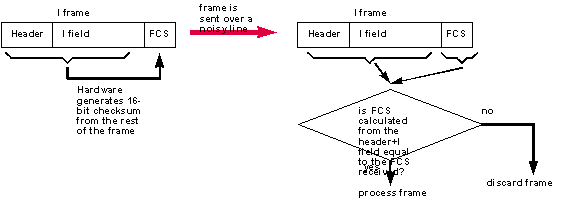There are a number of possible methods of error detection:

Parity bit on each character - This has the following disadvantages,

1. Uses 1 bit in every byte so only 7 bits are left for data, this means that it can only be used for ASCII characters without transparent 8-bit data.
2. An even number of errors will not be detected.
3. High overhead, ie 1 in 8 bits do not carry useful data.
4. There in no standard about whether even or odd parity is used.

* Longtitudinal parity byte - last byte in a block of data contains a parity byte, this still has the disadvantage that an even number of errors in any row would not be detected.

* Hamming codes - The principle of parity checks can be extended by adding more bits this can also allow errors to be corrected

without being re-transmitted. This has the disadvantage of using more bits and therefore being even less efficient.

slide LNK13 - error correction

## 11.2 - Polynomial code

Also known as a cyclic redundancy code (CRC) or frame check sequence (FCS). This uses a different form of arithmetic, the rules are the same as binary arithmetic except that there are no carries.

polynomial addition and subtraction thus become the same as 'exclusive or' on each corresponding pair of bits.

polynomial division is therefore done as follows:

1 1 0 0 0 0 1 0 1 0 <--- result

----------------------------

1 0 0 1 1 ) 1 1 0 1 0 1 1 0 1 1 0 0 0 0

1 0 0 1 1 | . . . . . . . .

--------- ?

1 0 0 1 1 . . . . . . . .

1 0 0 1 1 | . . . . . . .

--------- ?

0 0 0 0 1 . . . . . . .

0 0 0 0 0 | . . . . . .

--------- ?

0 0 0 1 0 . . . . . .

0 0 0 0 0 | . . . . .

--------- ?

0 0 1 0 1 . . . . .

0 0 0 0 0 | . . . .

--------- ?

0 1 0 1 1 . . . .

0 0 0 0 0 | . . .

--------- ?

1 0 1 1 0 . . .

1 0 0 1 1 | . .

--------- ?

0 1 0 1 0 . .

0 0 0 0 0 | .

--------- ?

1 0 1 0 0 .

1 0 0 1 1 |

--------- ?

0 1 1 1 0

0 0 0 0 0

---------

0 1 1 1 0 <---- remainder

in practice this can be computed by using a shift register as follows: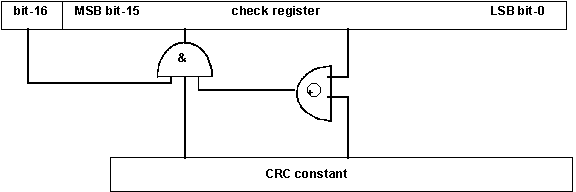This is normally built into the SDLC/HDLC chip.

## 11.3 - X25 link level Frame check sequence (FCS) field

The notation used to describe the FCS is based on the property of cyclic codes that a code vector such as 1000000100001 can be represented by a

12 5

polynomial P(x)=x + x + 1. The elements of an n-element code word are thus the coefficients of a polynomial of order n-1. In this application, these coefficients can have the value 0 or 1 and the polynomial operations are performed modulo 2. The polynomial representing the content of a frame is generated using the first bit received after the frame opening flag as the coefficient of the highest order term.

The FCS field shall be a 16-bit sequence. It shall be the ones

complement of the sum (modulo 2) of:

k 15 14 13 12 11 10 9 8 7 6 5

1) the remainder of x (x + x + x + x + x + x + x + x + x + x + x

4 3 2

+ x + x + x + x + 1)

16 12 5

divided (modulo 2) by the generator polynomial x + x + x + 1, where k is the number of bits in the frame existing between, but not including, the final bit of the opening flag and the first bit of the FCS, excluding bits inserted for transparency, and

2) the remainder of the division (modulo 2) by the generator

polynomial

16 12 5 16

x + x + x + 1 of the product of x by the content of the frame, existing between but not including, the final bit of the opening flag and the first bit of the FCS, excluding bits inserted for transparency.

As a typical implementation, at the transmitter, the initial content of the register of the device computing the remainder of the division is preset to all 1s and is then modified by division by the generator polynomial (as described above) on the address, control and information fields; the ones compliment of the resulting remainder is transmitted as the 16-bit FCS.

At the receiver, the initial content of the register of the device computing the remainder is preset to all 1s. The final remainder, after

16

multiplication by x and then division (modulo 2) by the

generator

polynomial 16 12 5 of the serial incoming protected

x + x + x + 1

bits and the

15 0

FCS, will be 0001110100001111 (x through x ,respectively) in the absence of transmission errors.

If you have lots of time and a BBC micro try writing a program to calculate the FCS.

Here is a start:

The 16-bit FCS is generated using polynomial arithmetic as follows:

%HFFFF + (REM(%HFFFF/gen)+REM(gen/frame))

where gen = generator polynomial = 1005 hexadecimal

%H = number is in hex

/ = polynomial division - not normal division

REM integers must contain 32 bits

CRCCONST = %H1005

DEF PROCinit

DEF bitarray(128):REM holds the string to be checked

checkreg = %HFFFF:REM as upto 128 8-bit numbers

pointer=1

length = 16

ENDPROC

DEF PROCexor (checkreg,crcconst)

checkreg = (checkreg AND %HFFFF) XOR crcconst

ENDPROC

DEF PROCbit

checkreg=checkreg * 2 + bitarray (pointer)

IF checkreg > %HFFFF THEN PROCexor

ENDPROC

DEF PROCdivremain

FOR pointer=1 TO length

PROCbit

NEXT

PRINT "result = ",checkreg

ENDPROC

# 13 - Bringing the link up

in LAPB:

The link can be brought up by either end by sending a SABM. The link can be taken down by either end by sending a DISC. Both DISCs and SABMs must be acknowledged by the other end with a UA Unlike other networks Public Network polls with DISCs when it is in the down state the first poll has the P-bit = 0 and following polls have the P-bit=1

slide EX 2 mk 2

## 13.1 - Example - Link setup

The example below is taken from a trace of a link being setup (it is in Hexadecimal), your task is to fill in the missing numbers:

Customer Network

----------------------------------------------------------------

/-------\

?------| 0 | 4 |-------

| 3 | 3 |

\-------/

/-------\

?------| 0 | 5 |-------

| 3 | 3 |

\-------/

/-------\

?------| 0 | 5 |-------

| 3 | 3 |

\-------/

/-------\

------| | |-------?

| | |

\-------/

/-------\

------| | |-------?

| | |

\-------/

/-------\

?------| | |-------

| | |

\-------/

13.2 - Slide EX 2 ____ _ _____ __ _

• This slide was made up from a printout from a halcyon (the halcyon screen is the same except it does not have the line with all the NNNNN's)
• each byte is represented by two hex digits above & below each other e.g.,

4

C = 01001100

• the display is read left to right / top to bottom
• both directions of transmission are displayed top is from host to network bottom is from network to host

the trace then continues in pairs like this down the screen

• the flags (7E) are suppressed

apart from the last flag in each frame

* the 16 byte FCS at the end of each frame is suppressed

13.3 - Example 2 - Answer ____ _ _______ _ _ ______

First frame - 03 EA 14 00 02 48 45 4C 4C 4F

- address = 03 = A - network to host so this is a command

- control = EA = 11101010

<->^<->^

| | | +-- this is an I frame

| | +---- N(S)=5

| +------ P=0

+-------- N(R)=7

- packet level is 14 00 02 48 45 4C 4C 4F

- FCS not displayed

Second frame - 01 CE 14 00 41

Address = 01 = B - host to network so this is a command

Control = CE = 11001110

<->^<->^

| | | +-- 0 so this is information frame

| | +---- N(S) = 7

| +------ P=0

+-------- N(R) = 6

Data = 14 00 41

FCS not displayed

Third frame - 01 01

- address = 01 = B - network to host so this is a response

- control = 01 = 00000001

<->^<-->

| | +--- this is an RR frame

| +------ P=0

+-------- N(R)=0

- RR frames do not have an I field

- FCS not displayed

slide EX 3 example 2 decoded slide EX 3 example 2 decoded slide EX 3 example 2 decoded

# 14 - HDLC/X.25 Information Transfer

## 14.1 - Sequence numbers

To be able to recover from line errors in either direction, with minimum slowing of throughput and without duplicating the data or getting the data in the wrong order is a very difficult thing to do.

To do this sequence numbers are used. these are 3-bit numbers in the control field of some frames, called:

N(S) pronounced N of S

N(R) pronounced N of R

Each time an I-frame is sent N(S) is incremented by one. Since the sequence numbers are carried by 3 binary digits the numbers cycle back to 0 after they get to 7: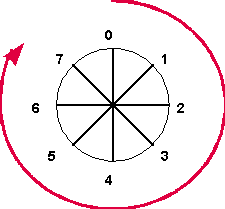N(S) in each direction is independent.

N(R) is used in the reverse direction to say what is the next

N(S) expected and to acknowledge all I frames up to that point.

At the link level there must not be more than 7 unacknowledged I frames at any time, this is known as the window size. If there are no unacknowledged I frames the window is said to be open, if there are 7 unacknowledged I frames the window is said to be closed.

## 14.2 - Simple case - data in one direction -

DTE DCE

---- SABM P=0 -------------------->

<--- UA F=0 -----------------------

---- I frame,P=0,N(S)=0,N(R)=0 --->

<--- RR frame,F=0,N(R)=1 ----------

---- I frame,P=0,N(S)=1,N(R)=0 --->

<--- RR frame,F=0,N(R)=2 ----------

---- I frame,P=0,N(S)=2,N(R)=0 --->

<--- RR frame,F=0,N(R)=3 ----------

---- I frame,P=0,N(S)=3,N(R)=0 --->

<--- RR frame,F=0,N(R)=4 ----------

---- I frame,P=0,N(S)=4,N(R)=0 --->

<--- RR frame,F=0,N(R)=5 ----------

---- I frame,P=0,N(S)=5,N(R)=0 --->

<--- RR frame,F=0,N(R)=6 ----------

---- I frame,P=0,N(S)=6,N(R)=0 --->

<--- RR frame,F=0,N(R)=7 ----------

---- I frame,P=0,N(S)=7,N(R)=0 --->

<--- RR frame,F=0,N(R)=0 ---------- | sequence numbers

| continue to

---- I frame,P=0,N(S)=0,N(R)=0 ---> | cycle round back

| to zero

<--- RR frame,F=0,N(R)=1 ----------

## 14.3 - Resetting sequence numbers

The sequence numbers keep incrementing as data is sent, the only thing that will reset the sequence numbers back to zero is a SABM-UA exchange.

When the link is already in the UP state, a SABM-UA exchange will not alter the state of the link and can be used to reset the sequence numbers to zero. This would only be necessary if one end lost track of its sequence numbers.

DTE DCE

---- I frame,P=0,N(S)=0,N(R)=0 --->

<--- RR frame,F=0,N(R)=1 ----------

---- I frame,P=0,N(S)=1,N(R)=0 --->

<--- RR frame,F=0,N(R)=2 ----------

---- SABM P=0 --------------------> | reset sequence

| numbers

<--- UA F=0 -----------------------

---- I frame,P=0,N(S)=0,N(R)=0 --->

<--- RR frame,F=0,N(R)=1 ----------

---- I frame,P=0,N(S)=1,N(R)=0 --->

<--- RR frame,F=0,N(R)=2 ----------

## 14.4 - Sequence numbers - continued

piggy backed acknowledgments:

DTE DCE

---- I frame,P=0,N(S)=0,N(R)=0 --->

<--- I frame,P=0,N(S)=0,N(R)=1 ----

---- I frame,P=0,N(S)=1,N(R)=0 --->

Multiple acknowledgments and closing window

DTE DCE

---- I frame,P=0,N(S)=0,N(R)=0 --->

---- I frame,P=0,N(S)=1,N(R)=0 --->

---- I frame,P=0,N(S)=2,N(R)=0 --->

---- I frame,P=0,N(S)=3,N(R)=0 --->

---- I frame,P=0,N(S)=4,N(R)=0 --->

---- I frame,P=0,N(S)=5,N(R)=0 --->

---- I frame,P=0,N(S)=6,N(R)=0 ---> | window is now

| closed - no more

| I frames can be

| sent until an

| acknowledgment

<--- RR frame,F=0,N(R)=7 ---------- | This RR frame

| acknowledges all

| of

| the above I frames

---- I frame,P=0,N(S)=7,N(R)=0 ---> | more frames can

| now be

sent

14.5 - Sequence numbers continued - line errors ____ _ ________ _______ _________ _ ____ ______

DTE DCE

---- I frame,P=0,N(S)=0,N(R)=0 ---> | receiver ignores

^ *** bad FCS **** | frames with fcs

| | errors

T1 timer |

| |

| |

v | no acknowledgment

---- I frame,P=1,N(S)=0,N(R)=0 ---> | so sender retransmits

| frame after T1 timer

| with P bit set to 1

<--- RR frame,F=1,N(R)=0 --------- | F bit set to 1 shows

| that RR is ack-ing

| retransmitted I

| frame.

Sequence numbers continued - line errors - alternate method used by Public Network ________ _______ _________ _ ____ ______ _ _________ ______ ____ __ ___

DCE (Public Network) DTE (Public Network)

---- I frame,P=0,N(S)=0,N(R)=0 ---> | receiver ignores

^ *** bad FCS **** | frames with fcs

| | errors

T1 timer |

| |

| |

v | no acknowledgment

---- RR frame,P=1,N(R)=0 ---> | so poll with

| command RR

|

<--- RR frame,F=1,N(R)=0 --------- | F bit set to 1 shows

| that RR is ack-ing

| command RR frame.

---- I frame,P=1,N(S)=0,N(R)=0 ---> | only when command

| RR is acknowledged

| is I frame re-

| transmitted

|

<--- RR frame,F=1,N(R)=0 --------- | F bit set to 1 shows

| that RR is ack-ing

| retransmitted I

| frame.

Sequence numbers - line errors in the middle of a sequence of I frames

DTE DCE

---- I frame,P=0,N(S)=0,N(R)=0 ---> | receiver ignores

*** bad FCS **** | frames with fcs

| errors

---- I frame,P=0,N(S)=1,N(R)=0 ---> | sender transmits the

| next frame before T1

| has expired

<--- REJ frame,F=0,N(R)=0 --------- | REJ used to request

| retransmission of

| frames starting with

| N(S)=0

---- I frame,P=1,N(S)=0,N(R)=0 ---> | so sender retransmits

| with P bit set to 1

<--- RR frame,F=1,N(R)=1 --------- | F bit set to 1 shows

| that RR is ack-ing

---- I frame,P=0,N(S)=1,N(R)=0 ---> | retransmitted I

| frame.

<--- RR frame,F=0,N(R)=2 ---------

14.6 - Sequence numbers - bad FCS in RR frame ____ _ ________ _______ _ ___ ___ __ __ _____

DTE DCE

---- I frame,P=0,N(S)=0,N(R)=0 ---> |

<--- RR frame,F=0,N(R)=1 --------- | sender ignores frame

| |

T1 timer |

| |

| |

v | no acknowledgment

---- I frame,P=1,N(S)=0,N(R)=0 ---> | so sender retransmits

| frame after T1 timer

| with P bit set to 1

<--- RR frame,F=1,N(R)=0 --------- | F bit set to 1 shows

| that RR is ack-ing

| retransmitted I

| frame.

## 14.7 - Busy State

If one end of the link is in danger of becoming congested, e.g. is running out of resources such as buffers, CPU time etc. it can "hold-off" the other end by sending an RNR, this is more immediate than waiting for the window to close.

note - unlike packet level RNRs, link level RNRs affect all calls over the link.

DTE DCE

---- I frame,P=0,N(S)=0,N(R)=0 --->

---- I frame,P=0,N(S)=1,N(R)=0 --->

---- I frame,P=0,N(S)=2,N(R)=0 --->

<--- RNR frame,F=0,N(R)=3 --------- | This RNR frame

| acknowledges all of

| the above I frames

| don't send any more

.

.

sender now waits for receiver to become free optionally the sender can poll with command RRs the receiver will respond with RNRs until it wishes to free its busy

.

<--- RR frame,F=0,N(R)=3 ---------- | This RR frame

| takes off the busy

---- I frame,P=0,N(S)=3,N(R)=0 ---> | more frames can

| now be sent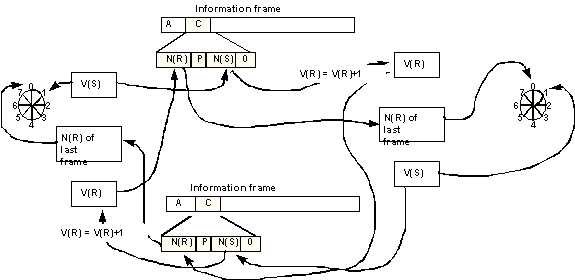Multilink (not to be confused with multiline) allows more than one physical link to be considered as one logical link.

It is a method of load sharing at level 2, it provides much better load sharing than multiline which is done at level 3 and can only share on a per call basis.

However multilink is horrendously complicated and is not yet supported by Public Network.

 Flag address control MLC <- 2bytes -> Information FCS Flag

1-4 5 6 7 8 9-16

MNS(S) V S R C MNL(S)

MNH(S) bits 9-12 of 12-bit multilink send sequence number MN(S)

MNL(S) bits 1-8 of 12-bit multilink send sequence number MN(S)

V void sequencing bit

S sequence check option bit

R MLP reset request bit

C MLP reset confirmation

## Practical L1 - Decoding Frames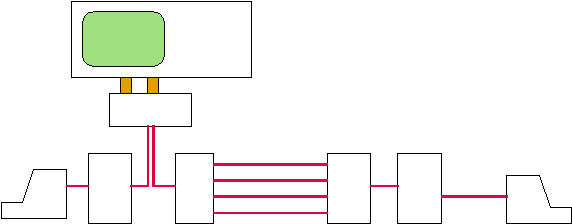Practical L2 - simulating frames using Tekelec simulator

Procedure

1 - connect up the equipment as shown below:

---\ /-------------------------\ /-----------\ ---

/--/ |---| |-----------------| port|---| \---

\------/---| |-----------------|trunk |---|-------|

Texas /--| | | | terminal

Printer |/-| | | |

|| \-------------------------/ \-----------/

|| Tekelec TE92 or Chameleon PAD(DTE)

||

|| /-------------------\

|\-| |

\--| Keyboard |

\-------------------/

2 - Connect and switch on mains power to all equipment.

3 - Set up Tekelec as simulator

and bring the link up (SLON,SLG4,SPOF)

4 - Use the Tekelec to make a call and send some data, you will

have to work out the packets and send them in hexadecimal, when

you have worked out what to send thene PH followed by pairs of

hexadecimal digits with no spaces in between -

e.g., PH14060B0E2342192010064000

5 - Try sending all the other types of packet.

Sample printouts from HP ______ _________ ____ __

An example of normal data transfer, with level 2 decode, the I-

field data here is not meaningful, in practice if you need to

read this use another type of decode or display in hex.

Hewlett - Packard 4953A Protocol Analyzer

DTE DCE

ADDRESS TYPE N(S) P/F N(R) INFO FCS ADDRESS TYPE N(S) P/F N(R) INFO FCS

0 Info 5 0 7 D)Sl G

1 2 X

0 Info 6 0 7 D)! G

1 2

0 Info 7 0 5 D*! G

3 2

0 RR 0 0 G

3

0 Info 7 0 0 D*E G

1 2 4

0 Info 0 0 5 D(A G

3 2 1

0 RR 0 6 G

1

0 RR 0 1 G

3

0 Info 0 0 1 D(lRD G

1 2 S4

0 REJ 0 6 G

1

0 Info 1 0 1 DCAFN G

1 2N6FU

0 Info 6 0 1 D)! G

1 2

0 RR 0 6 G

1

0 Info 7 0 1 D*E G

1 2 4

BLOCK NUMBER = 40

DTE DCE

ADDRESS TYPE N(S) P/F N(R) INFO FCS ADDRESS TYPE N(S) P/F N(R) INFO FCS

0 RR 0 6 G

1

0 RR 0 7 G

1

0 Info 0 0 1 D(lRD G

1 2 S4

0 RR 0 0 G

1

0 Info 1 0 1 DCAFN G

1 2N6FU

0 Info 2 0 1 DCAFN G

1 2N8FU

0 RR 0 1 G

1

0 RR 0 2 G

1

0 RR 0 3 G

1

0 Info 1 0 3 DDa G

3 24

0 RR 0 2 G

3

0 Info 3 0 2 DDARD G

1 248S4

0 RR 0 4 G

1

0 Info 2 0 4 DBE G

3 2L1

0 RR 0 3 G

3

0 Info 4 0 3 DB RD G

1 2L S4

BLOCK NUMBER = 40

Another example printout: _______ _______ _________

Hewlett - Packard 4953A Protocol Analyzer

ADDRESS TYPE N(S) P/F N(R) QD MOD LCN TYPE P(S) M P(R) DATA FCS

0 Info 2 0 4 00 8 07D Call E241212724121

1 E327003732700

0700800533333330044444440 G

57210063907123406D1C3FCDD

0 RR 0 3

1

G

0 Info 4 0 3 00 8 07D Call Acc E241212724121

3 E327003732700

070400400A2422102FFFFFFFFFFFFFFFF0445452222415100E000544442222412107E22247 G

57632227703230034FFFFFFFFFFFFFFFF5812D70003270040000641E4D0003290064000025

0 Info 5 0 3 00 8 07D Data 0 0 0 0222222222444

3 7A00AAAA0025C

4455255442444254424454545222222 G

613403353092D03E10714571900AAAA

0 FRMR 0 680

3 864

G

0 SABM 0

3

G

0 FRMR 0 680

3 864

G

0 SABM 0

3

G

BLOCK NUMBER = 65

 metadata block see also: Correspondence about this page Book Shop - Further reading. Where I can, I have put links to Amazon for books that are relevant to the subject, click on the appropriate country flag to get more details of the book or to buy it from them.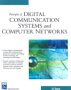Principles of Digital Communication systems and computer networks. - Designed as a textbook for courses on digital communication systems, data communication, computer networks and mobile computing. Commercial Software Shop Where I can, I have put links to Amazon for commercial software, not directly related to the software project, but related to the subject being discussed, click on the appropriate country flag to get more details of the software or to buy it from them.Palm Treo 650 GSM/GPRS Smartphone

This site may have errors. Don't use for critical systems.